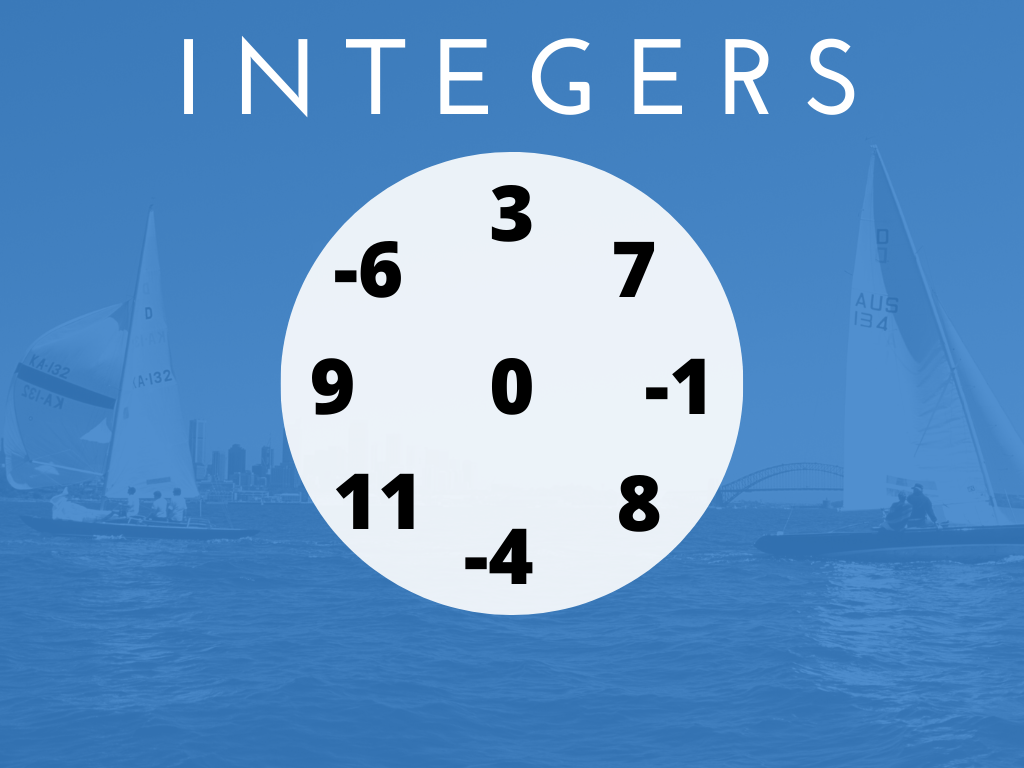# Integers Class 7th

## Introduction

We studied natural numbers, whole numbers, and integers in the previous class. We know that the collection of all the positive and negative numbers along 0 are called integers. We can also say that integers are the set of whole numbers and negative numbers. In class 7th, we will study more about integers like properties of integers, multiplication, and division of integers with the help of different types of examples which we had not studied in the previous class.

### Definition

All the positive numbers and negative numbers including 0 are known as integers. Integers are denoted by Z.

Examples – ………………-5, -4, -3, -2, -1, 0, 1, 2, 3, 4, 5……………..

### Representation and Operations of Integers on the Number Line

We have practiced the representation and operations of integers on the number line in class 6th. Let’s recall these with the help of some examples.

Example 1) Represent the following integers on the number line.

a) 5       b) -4       c) 2       d) 0       e) -3

Solution –

Example 2) Add the following integers on the number line.

a) 2 and 3      b) -1 and 5     c) 4 and -6     d) -2 and -3

Solution – a) 2 and 3

Therefore, the addition of 2 and 3

2 + 3 = 5              Ans.

b) -1 and 5

Therefore, the addition of -1 and 5

-1 + 5 = 4           Ans.

c) 4 and -6

Therefore, the addition of 4 and -6

4 + (-6) = -2        Ans.

d) -2 and -3

Therefore, the addition of -2 and -3

-2 + (-3) = -5          Ans.

In the addition of integers on the number line, we have to remember the points given below.

1) When we add two positive integers, we move towards the right side on the number line. See the addition of 2 and 3 in the above example.

2) When we add a negative and a positive integer, first we move towards the left side and after that, we move towards the right side on the number line. See the addition of -1 and 5 in the above example.

3) When we add a positive and a negative integer, first we move towards the right side and after that, we move towards the left side on the number line. See the addition of 4 and -6 in the above example.

4) When we add two negative integers, we move towards the left side on the number line. See the addition of -2 and -3 in the above example.

### Division of Integers

Integers Class 7th in Hindi